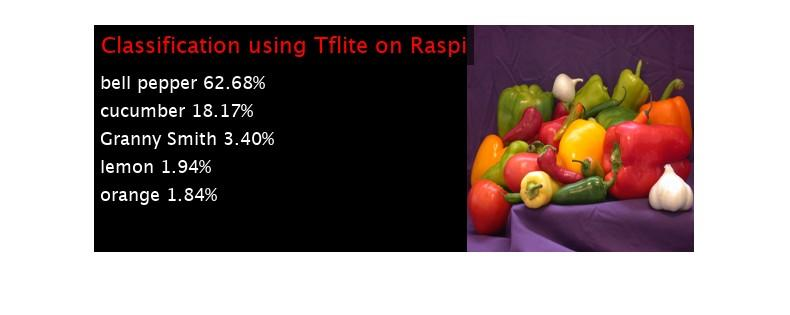# Generate Code for TensorFlow Lite (TFLite) Model and Deploy on Raspberry Pi

This example shows how to generate code that performs inference by using a Tensorflow™ Lite model and deploy the code on Raspberry Pi™ hardware. This example uses a pretrained TensorFlow Lite model for the image classification network Mobilenet-V1 that is available on the TensorFlow webpage for Hosted models. In example, you use the `codegen` (MATLAB Coder) command to generate a PIL MEX function that runs the generated executable on the target hardware. This workflow can be used for both `int8` and `float` TensorFlow Lite models.

This example is supported for host Windows® and Linux® platforms.

### Third-Party Prerequisites

• Raspberry Pi hardware

• TensorFlow Lite library (on the target ARM® hardware)

• Pretrained TensorFlow Lite Model

Run this script to download the image classification network Mobilenet-V1 from the URL mentioned below.

```if ~exist("model.tgz","file") disp('Downloading 5 MB Mobilenet-V1 model file...'); url = "https://storage.googleapis.com/download.tensorflow.org/models/mobilenet_v1_2018_02_22/mobilenet_v1_0.5_224.tgz"; websave("model.tgz",url); untar("model.tgz"); end```

### The `tflite_predict` Entry-Point Function

The `loadTFLiteModel` function loads the Mobilenet-V1 model into a `TFLiteModel` object. The properties of this object contain information about the model such as the number and size of inputs and outputs of the model.

```net = loadTFLiteModel('mobilenet_v1_0.5_224.tflite'); disp(net); TFLiteModel with properties: ModelName: 'mobilenet_v1_0.5_224.tflite' NumInputs: 1 NumOutputs: 1 InputSize: {[224 224 3]} OutputSize: {[1001 1]} NumThreads: 8 Mean: 127.5000 StandardDeviation: 127.5000 ```

In this example, you generate code for the entry-point function `tflite_predict.m`. This function loads the Mobilenet-V1 model into a persistent network object by using the `loadTFLiteModel` function. To optimize performance, after creating the network object, set the `NumThreads` property based on the number of threads available on your hardware board.

The `tflite_predict` function performs prediction by passing the network object to the `predict` function. Subsequent calls to this function reuse this persistent object.

`type tflite_predict.m`
```function out = tflite_predict(in) persistent net; if isempty(net) net = loadTFLiteModel('mobilenet_v1_0.5_224.tflite'); % To optimize performance, set NumThreads property based on the number % of threads available on the hardware board net.NumThreads = 4; end out = net.predict(in); end ```

On the Raspberry Pi hardware, set the environment variable TFLITE_PATH to the location of the TensorFlow Lite library. For more information on how to build the TensorFlow Lite library and set the environment variables, see Prerequisites for Deep Learning with TensorFlow Lite Models.

### Generate PIL MEX Function

#### Create Code Configuration Object

To generate a PIL MEX function for a specified entry-point function, create a code configuration object for a static library and set the verification mode to `'PIL'`. Set the target language to C++.

```cfg = coder.config('lib', 'ecoder', true); cfg.TargetLang = 'C++'; cfg.VerificationMode = 'PIL'; ```

#### Set Up Connection with Raspberry Pi

Use the MATLAB Support Package for Raspberry Pi Hardware function `raspi` to create a connection to the Raspberry Pi.

In the following code, replace:

• raspiname with the name of your Raspberry Pi board

```r = raspi('raspiname','username','password'); ```

#### Configure Code Generation Hardware Parameters for Raspberry Pi

Create a `coder.hardware` (MATLAB Coder) object for Raspberry Pi and attach it to the code generation configuration object.

```hw = coder.hardware('Raspberry Pi'); cfg.Hardware = hw; ```

#### Copy TensorFlow Lite model to Target Hardware

Copy the TensorFlow Lite model to the Raspberry Pi board. On the hardware board, set the environment variable TFLITE_MODEL_PATH to the location of the TensorFlow Lite model. For more information on setting environment variables, see Prerequisites for Deep Learning with TensorFlow Lite Models.

In the following command, replace `targetDir` with the destination folder of TensorFlow Lite model on the Raspberry Pi board.

```r.putFile('mobilenet_v1_0.5_224.tflite',targetDir) ```

#### Generate PIL MEX

On the host platform, run the `codegen` command to generate a PIL MEX function `tflite_predict_pil`.

```codegen -config cfg tflite_predict -args ones(224,224,3,'single') ```

### Run Generated PIL MEX

Read the input image by using `imread`. Resize the input to reqired input size of the network.

```I = imread('peppers.png'); I1 = single(imresize(I,[224,224])); ```

Run the generated PIL MEX by passing the resized input.

```predictionScores = tflite_predict_pil(I1); ```

Map the prediction scores onto the image.

```DisplayPredsonImage(predictionScores, I); ```